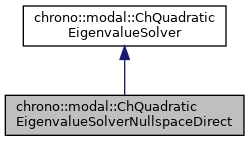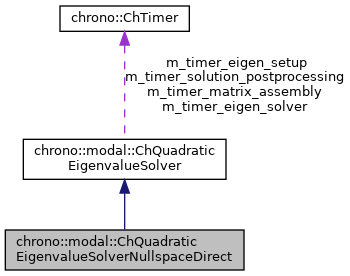## Description

Solves the eigenvalue problem with a direct method: first does LU factorization of Cq jacobians to find the null space, then solves the problem using the direct Eigen::EigenSolver.

Note: since intermediate dense matrices are built, the performance is acceptable only for small-sized problems. Note: since the method is direct, all eigenvalues are computed, regardless of n_modes, but only lower n_modes are returned.

#include <ChEigenvalueSolver.h>[legend][legend]

## Public Member Functions

virtual bool Solve (const ChSparseMatrix &M, const ChSparseMatrix &R, const ChSparseMatrix &K, const ChSparseMatrix &Cq, ChMatrixDynamic< std::complex< double >> &V, ChVectorDynamic< std::complex< double >> &eig, ChVectorDynamic< double > &freq, ChVectorDynamic< double > &damping_ratio, ChEigenvalueSolverSettings settings=0) const override
Solve the quadratic eigenvalue problem (lambda^2*M + lambda*R + K)*x = 0 s.t. More...Public Member Functions inherited from chrono::modal::ChQuadraticEigenvalueSolver
double GetTimeMatrixAssembly () const
Get cumulative time for matrix assembly.

double GetTimeEigenSetup () const
Get cumulative time eigensolver setup.

double GetTimeEigenSolver () const
Get cumulative time eigensolver solution.

double GetTimeSolutionPostProcessing () const
Get cumulative time for post-solver solution postprocessing.Protected Attributes inherited from chrono::modal::ChQuadraticEigenvalueSolver
ChTimer m_timer_matrix_assembly
timer for matrix assembly

ChTimer m_timer_eigen_setup
timer for eigensolver setup

ChTimer m_timer_eigen_solver
timer for eigensolver solution

ChTimer m_timer_solution_postprocessing
timer for conversion of eigensolver solution

## ◆ Solve()

 bool chrono::modal::ChQuadraticEigenvalueSolverNullspaceDirect::Solve ( const ChSparseMatrix & M, const ChSparseMatrix & R, const ChSparseMatrix & K, const ChSparseMatrix & Cq, ChMatrixDynamic< std::complex< double >> & V, ChVectorDynamic< std::complex< double >> & eig, ChVectorDynamic< double > & freq, ChVectorDynamic< double > & damping_ratio, ChEigenvalueSolverSettings settings = 0 ) const
overridevirtual

Solve the quadratic eigenvalue problem (lambda^2*M + lambda*R + K)*x = 0 s.t.

Cq*x = 0 If n_modes=0, return all eigenvalues, otherwise only the first lower n_modes.

Parameters
 M input M matrix R input R matrix K input K matrix Cq input Cq matrix of constraint jacobians V output matrix with eigenvectors as columns, will be resized eig output vector with complex eigenvalues (real part not zero if some damping), will be resized freq output vector with n frequencies [Hz], as f=w/(2*PI), will be resized. damping_ratio output vector with n damping rations r=damping/critical_damping. settings optional: settings for the solver, or n. of desired lower eigenvalues. If =0, return all eigenvalues.